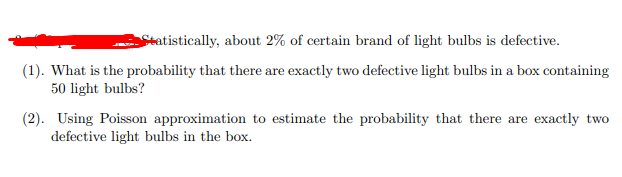# Question Solved1 AnswerStatistically, about 2% of certain brand of light bulbs is defective. (1). What is the probability that there are exactly two defective light bulbs in a box containing 50 light bulbs? (2). Using Poisson approximation to estimate the probability that there are exactly two defective light bulbs in the box.XMIBCU The Asker · Probability and StatisticsTranscribed Image Text: Statistically, about 2% of certain brand of light bulbs is defective. (1). What is the probability that there are exactly two defective light bulbs in a box containing 50 light bulbs? (2). Using Poisson approximation to estimate the probability that there are exactly two defective light bulbs in the box.
More
Transcribed Image Text: Statistically, about 2% of certain brand of light bulbs is defective. (1). What is the probability that there are exactly two defective light bulbs in a box containing 50 light bulbs? (2). Using Poisson approximation to estimate the probability that there are exactly two defective light bulbs in the box.